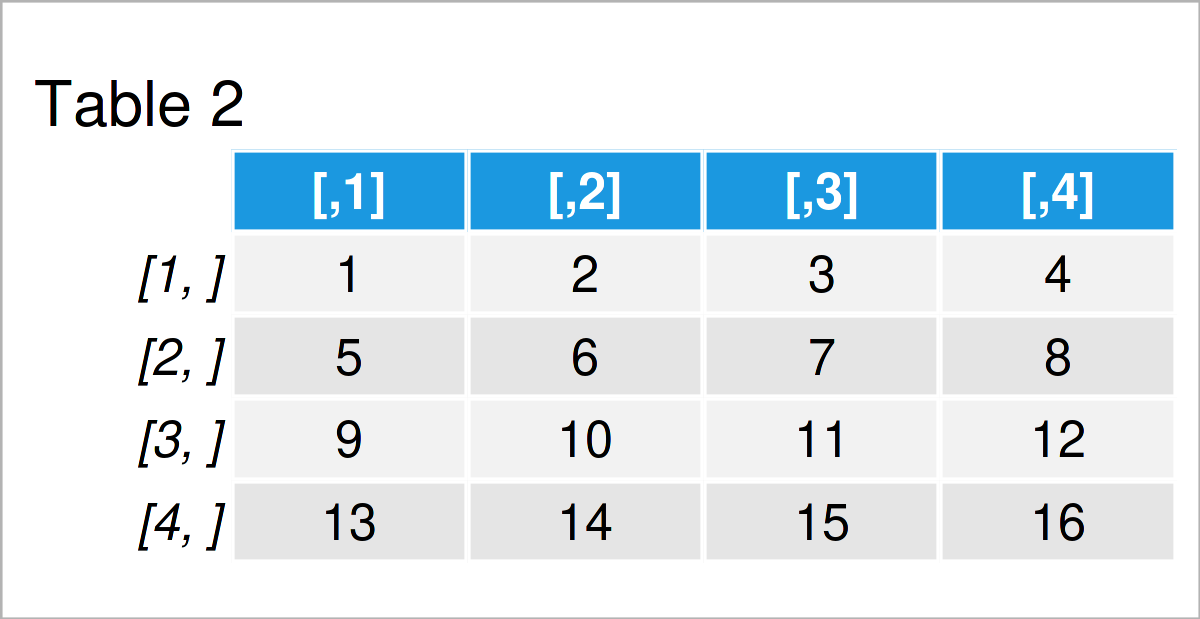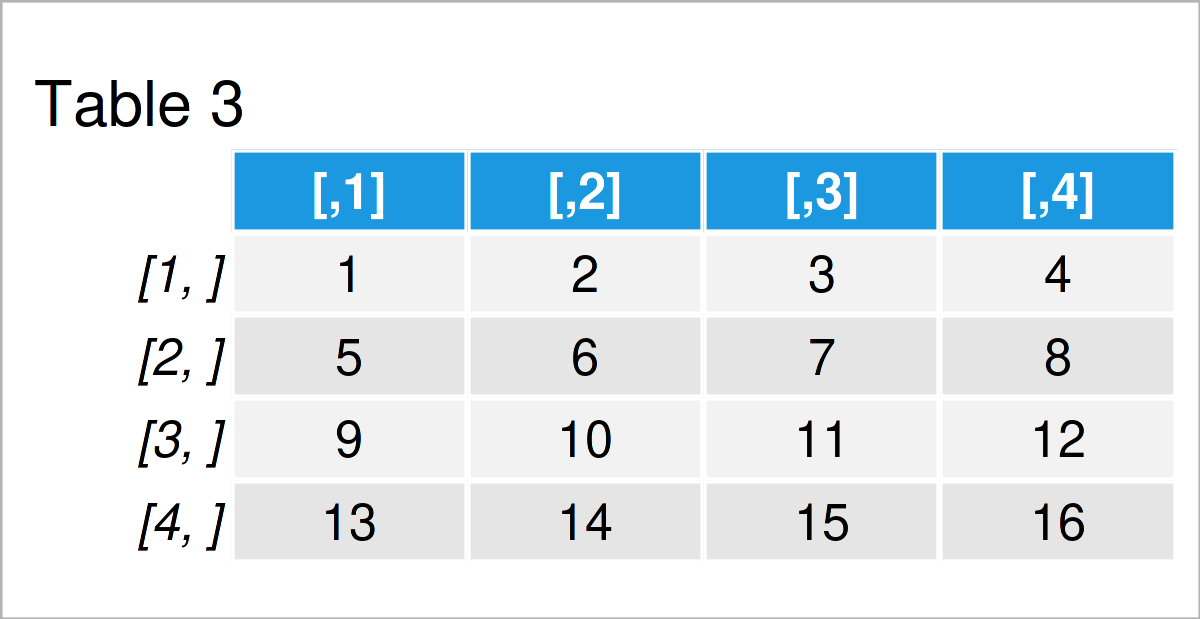# identity Function in R (2 Examples)

This tutorial illustrates how to return a data object using the identity function in the R programming language.

The article will consist of this:

Let’s get started!

## Example 1: Basic Application of identity() Function

In Example 1, I’ll explain how to use the identity function to return a data object.

For this, we first have to create a data object in R:

```x <- 1:5                                   # Create vector object
x                                          # Print vector object
#  1 2 3 4 5```

The previous output of the RStudio console shows that our data object is a vector containing five integer elements.

Let’s apply the identity function to our data object:

```identity(x)                                # Apply identity function to vector
#  1 2 3 4 5```

As you can see, the identity function has just returned its argument, i.e. our vector object.

So far, so good – But why should we even use the identity function? That’s what I’m going to show next.

## Example 2: Use identity() Function within Other Functions

In practice, the identity function is often used within other functions. Generally speaking: In functional programming languages, functions are often passed as arguments to other functions.

Let’s illustrate that with another example. First, we have to create some example data:

```my_mat <- matrix(1:16, ncol = 4)           # Create example matrix
my_mat                                     # Print example matrix```In Table 1 it is shown that we have created a matrix object containing four rows and four columns.

Next, we might use the apply and identity functions to transpose our matrix:

```my_mat_t1 <- apply(my_mat, 1, identity)    # Use identity function within apply
my_mat_t1                                  # Print transposed matrix```Table 2 shows the output of the previous R programming syntax: A transposed matrix. In the previous R code, we had to use the identity function, since we had to specify a function argument (i.e. FUN) within the apply function.

Obviously, this task could be done with the t function as well:

```my_mat_t2 <- t(my_mat)                     # Use t function for comparison
my_mat_t2                                  # Print transposed matrix```As shown in Table 3, the previous code has created the same transposed matrix with a simpler syntax. However, this example has shown why it is sometimes needed to rely on a function that just returns the values of its input argument.

## Video, Further Resources & Summary

In case you need more explanations and examples why the identity function is useful, you may check out this thread on Stack Overflow. The code of this tutorial is also partly based on this discussion.

Furthermore, you may want to read the other articles on statisticsglobe.com.

You have learned in this article how to apply the identity function in R programming. If you have additional questions, don’t hesitate to let me know in the comments section.

Subscribe to the Statistics Globe Newsletter